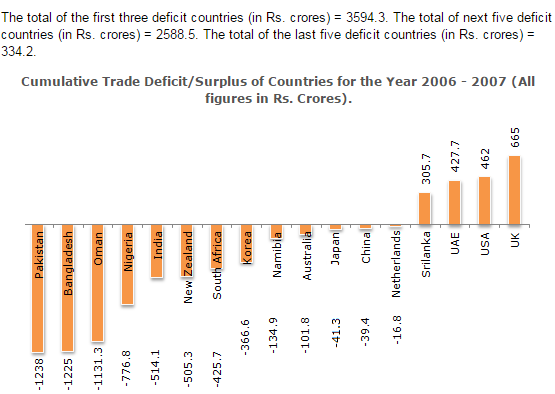Direction1.

The ratio between the difference between the highest and the lowest of the surplus countries to the difference between Bangladesh and Oman is ?

 A. 3.44 B. 2.96 C. 4.5 D. 3.83
Answer:D
Explanation: The required answer will be equal to 360/94 = 3.829.

2.

The average of the total deficit of the middle five deficit is closest to the deficit of which country ?

 A. Korea B. South Africa C. UAE D. None of these
Answer:A
Explanation: The middle five deficit countries are India, New Zealand, South Africa, Korea and Namibia. Their average deficit is (1946.6/5) = 389.32. Korea's deficit is close to this value.

3.

The state whose surplus is nearly equal to the average of the four surplus countries is

 A. Sri Lanka B. UAE C. USA D. UK
Answer:C
Explanation: The average trade surplus of the four countries is 1860.4/4 = 465.1. Of the four options, USA has the closest value.

4.

The ratio of the deficit of the first five deficit countries to the overall deficit of all the deficit countries is nearly equal to ?

 A. 0.72 B. 0.75 C. 0.8 D. 0.85
Answer:B
Explanation: The required answer is (4885.2/6517) = 0.7496

5.

The net total deficit/surplus is equal to ?

 A. 4656.6 surplus B. 4656.6 deficit C. 3836.5 deficit D. None of these
Answer:B
Explanation: Adding all surpluses and subtracting all deficits will give an overall value of deficit of 4656.6.